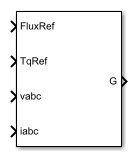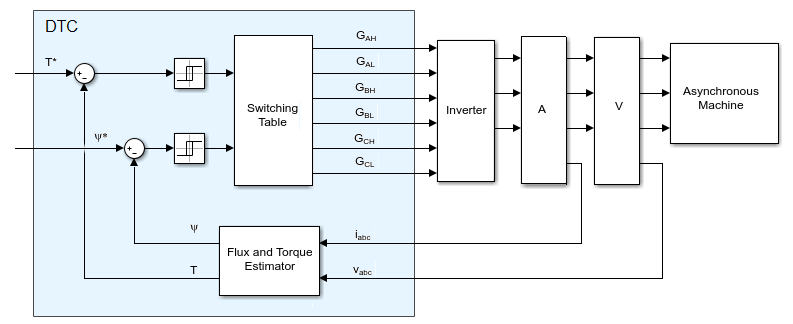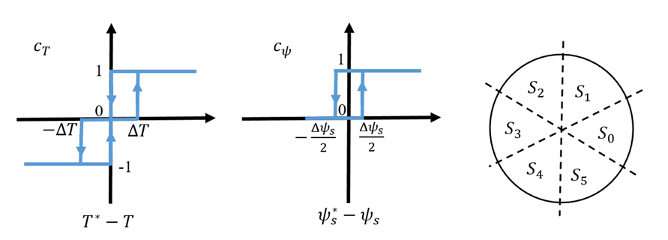# Induction Machine Direct Torque Control

Induction machine DTC

•Libraries:
Simscape / Electrical / Control / Induction Machine Control

## Description

The Induction Machine Direct Torque Control block implements an induction machine direct torque control (DTC) structure. The figure shows the equivalent circuit for the block.### Equations

To estimate the torque and flux, the Induction Machine Direct Torque Control block discretizes the machine voltage equations in the stationary ɑβ reference frame using the backward Euler method. The discrete-time equations for stator fluxes in the ɑβ frame are:

`${\psi }_{\alpha }=\left({v}_{\alpha }-{i}_{\alpha }{R}_{s}\right)\frac{{T}_{s}z}{z-1}$`

and

`${\psi }_{\beta }=\left({v}_{\beta }-{i}_{\beta }{R}_{s}\right)\frac{{T}_{s}z}{z-1}$`

where:

• vɑ is ɑ-axis voltage.

• iɑ is ɑ-axis current.

• Rs is the stator resistance.

• Ψɑ is the ɑ-axis stator flux.

• vβ is β-axis voltage.

• iβ is β-axis current.

• Ψβ is the β-axis stator flux.

The block calculates the torque and flux as:

`$T=\frac{3p}{2}\left({\psi }_{\alpha }{i}_{\beta }-{\psi }_{\beta }{i}_{\alpha }\right)$`

and

`${\psi }_{s}=\sqrt{{\psi }_{\alpha }^{2}+{\psi }_{\beta }^{2}}$`

where:

• p is the number of pole pairs.

• Ψs is the stator flux.

To detect flux and torque estimation errors, the block uses hysteresis comparators. The figure shows hysteresis comparators and the associated switching sectors.The table shows the optimum switching for an inverter high-side system.

cΨ, cTS(θ)S0S1S2S3S4S5
cΨ = 1cT = 1`1, 1, 0``0, 1, 0``0, 1, 1``0, 0, 1``1, 0, 1``1, 0, 0`
cT = 0`1, 1, 1``0, 0, 0``1, 1, 1``0, 0, 0``1, 1, 1``0, 0, 0`
cT = -1`1, 0, 1``1, 0, 0``1, 1, 0``0, 1, 0``0, 1, 1``0, 0, 1`
cΨ = 0cT = 1`0, 1, 0``0, 1, 1``0, 0, 1``1, 0, 1``1, 0, 0``1, 1, 0`
cT = 0`0, 0, 0``1, 1, 1``0, 0, 0``1, 1, 1``0, 0, 0``1, 1, 1`
cT = -1`0, 0, 1``1, 0, 1``1, 0, 0``1, 1, 0``0, 1, 0``0, 1, 1`

### Assumptions and Limitations

• The power inverter dead times are not considered. For hardware implementation, add the dead time externally.

## Ports

### Input

expand all

Reference stator flux.

Data Types: `single` | `double`

Reference torque.

Data Types: `single` | `double`

Stator phase voltages.

Data Types: `single` | `double`

Stator phase currents.

Data Types: `single` | `double`

### Output

expand all

Inverter gate pulses. The block does not consider any dead time.

Data Types: `single` | `double`

## Parameters

expand all

Resistance of the machine stator.

Number of machine pole pairs.

Total bandwidth distributed symmetrically around the flux set point.

Total bandwidth distributed symmetrically around the set point.

Time, in s, between consecutive block executions. During execution, the block produces outputs and, if appropriate, updates its internal state. For more information, see What Is Sample Time? and Specify Sample Time.

If this block is inside a triggered subsystem, inherit the sample time by setting this parameter to `-1`. If this block is in a continuous variable-step model, specify the sample time explicitly using a positive scalar.

 Takahashi, I., and T. Noguchi. "A New Quick-Response and High-Efficiency Control Strategy of an Induction Motor." IEEE Transactions on Industry Applications. Vol. IA-22, Number 5, 1986, pp. 820 - 827.